# SSAT Upper Level Math : How to find the fractional equivalent of a decimal

## Example Questions

2 Next →

### Example Question #261 : Rational Numbers

Express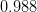as a fraction.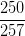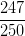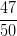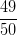Explanation:

Write the decimal divided by.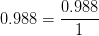Multiply the numerator and denominator by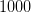to get rid of the decimal, then simplify.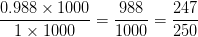### Example Question #11 : How To Find The Fractional Equivalent Of A Decimal

Find the fractional equivalent of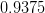.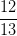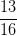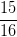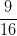Explanation:

Write the decimal divided by.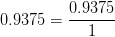Multiply the numerator and denominator by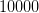to get rid of the decimal, then simplify.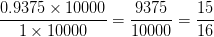### Example Question #263 : Rational Numbers

Find the fractional equivalent of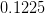.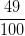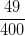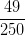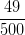Explanation:

Write the decimal divided by.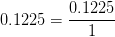Multiply the numerator and denominator byto get rid of the decimal, then simplify.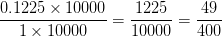### Example Question #264 : Rational Numbers

Find the fractional equivalent of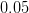.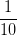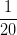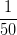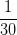Explanation:

Write the decimal divided by.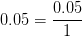Multiply the numerator and denominator by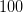to get rid of the decimal, then simplify.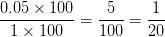### Example Question #265 : Rational Numbers

What is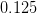as a fraction?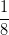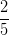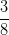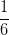Explanation:

Write the decimal divided by.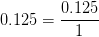Multiply the numerator and denominator byto get rid of the decimal, then simplify.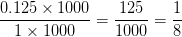### Example Question #266 : Rational Numbers

Convert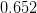into a fraction.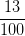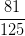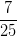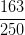Explanation:

Write the decimal divided by.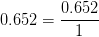Multiply the numerator and denominator byto get rid of the decimal, then simplify.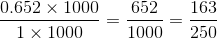2 Next →